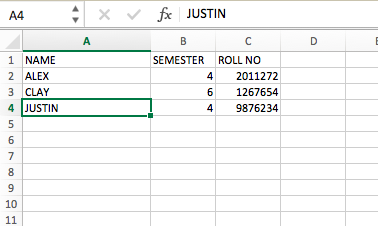# Reading an excel file using Python

• Difficulty Level : Easy
• Last Updated : 19 Feb, 2022

Using xlrd module, one can retrieve information from a spreadsheet. For example, reading, writing or modifying the data can be done in Python. Also, the user might have to go through various sheets and retrieve data based on some criteria or modify some rows and columns and do a lot of work.

xlrd module is used to extract data from a spreadsheet.

NOTE: xlrd has explicitly removed support for reading xlsx sheets.

Command to install xlrd module :

`pip install xlrd`

Input File:Code #1:  Extract a specific cell

## Python3

 `# Reading an excel file using Python` `import` `xlrd`   `# Give the location of the file` `loc ``=` `(``"path of file"``)`   `# To open Workbook` `wb ``=` `xlrd.open_workbook(loc)` `sheet ``=` `wb.sheet_by_index(``0``)`   `# For row 0 and column 0` `print``(sheet.cell_value(``0``, ``0``))`

Output :

`'NAME'`

Code #2: Extract the number of rows

## Python3

 `# Program to extract number` `# of rows using Python` `import` `xlrd`   `# Give the location of the file` `loc ``=` `(``"path of file"``)`   `wb ``=` `xlrd.open_workbook(loc)` `sheet ``=` `wb.sheet_by_index(``0``)` `sheet.cell_value(``0``, ``0``)`   `# Extracting number of rows` `print``(sheet.nrows)`

Output :

`4`

Code #3: Extract the number of columns

## Python3

 `# Program to extract number of` `# columns in Python` `import` `xlrd`   `loc ``=` `(``"path of file"``)`   `wb ``=` `xlrd.open_workbook(loc)` `sheet ``=` `wb.sheet_by_index(``0``)`   `# For row 0 and column 0` `sheet.cell_value(``0``, ``0``)`   `# Extracting number of columns` `print``(sheet.ncols)`

Output :

`3`

Code #4 : Extracting all columns name

## Python3

 `# Program extracting all columns` `# name in Python` `import` `xlrd`   `loc ``=` `(``"path of file"``)`   `wb ``=` `xlrd.open_workbook(loc)` `sheet ``=` `wb.sheet_by_index(``0``)`   `# For row 0 and column 0` `sheet.cell_value(``0``, ``0``)`   `for` `i ``in` `range``(sheet.ncols):` `    ``print``(sheet.cell_value(``0``, i))`

Output :

```NAME
SEMESTER
ROLL NO```

Code #5: Extract the first column

## Python3

 `# Program extracting first column` `import` `xlrd`   `loc ``=` `(``"path of file"``)`   `wb ``=` `xlrd.open_workbook(loc)` `sheet ``=` `wb.sheet_by_index(``0``)` `sheet.cell_value(``0``, ``0``)`   `for` `i ``in` `range``(sheet.nrows):` `    ``print``(sheet.cell_value(i, ``0``))`

Output :

```NAME
ALEX
CLAY
JUSTIN```

Code #6: Extract a particular row value

## Python3

 `# Program to extract a particular row value` `import` `xlrd`   `loc ``=` `(``"path of file"``)`   `wb ``=` `xlrd.open_workbook(loc)` `sheet ``=` `wb.sheet_by_index(``0``)`   `sheet.cell_value(``0``, ``0``)`   `print``(sheet.row_values(``1``))`

Output :

`['ALEX', 4.0, 2011272.0]`

My Personal Notes arrow_drop_up
Recommended Articles
Page :